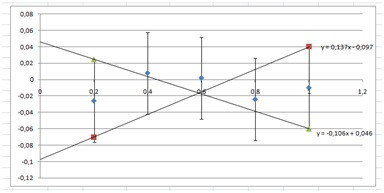# Data analysis 1, linear relations, Zooming in, but not through the origin

Up a level : IB Physics
Previous page : Data analysis 1, linear relations, proportionalities, Zooming in
Next page : Data analysis, part 2, more linear DataZooming in, but not through the origin

This is continuing the analysis of the data the Excel file analysis1 V2, in the leaf The Data, 2 that we started to analyse in the previous page.

How about if we do not know it goes through the origin? We can then add points to the last column:The values were chosen to make the slope as small or big as possible. The plot would now look like:The slopes are 0.137 and 0.106. That gives us  0±0.2. The “y”-intercepts are 0.046 and –0.097, that gives us –0.03±0.08.

This will finally give us

R=(1.4±0.2)L –0.03±0.08

The difference between the two cases, the previous analysis and this one,  is if you can assume it is a proportionality (goes through the origin) or not.

As you can see the result from this analysis is consistent with the result from the previous one, R=(1.41±0.05)L. The value zero is in the range –0.03±0.08, and 1.41±0.05 is within the range 1.4±0.2.Up a level : IB Physics
Previous page : Data analysis 1, linear relations, proportionalities, Zooming in
Next page : Data analysis, part 2, more linear DataLast modified: Jan 7, 2017 @ 15:06